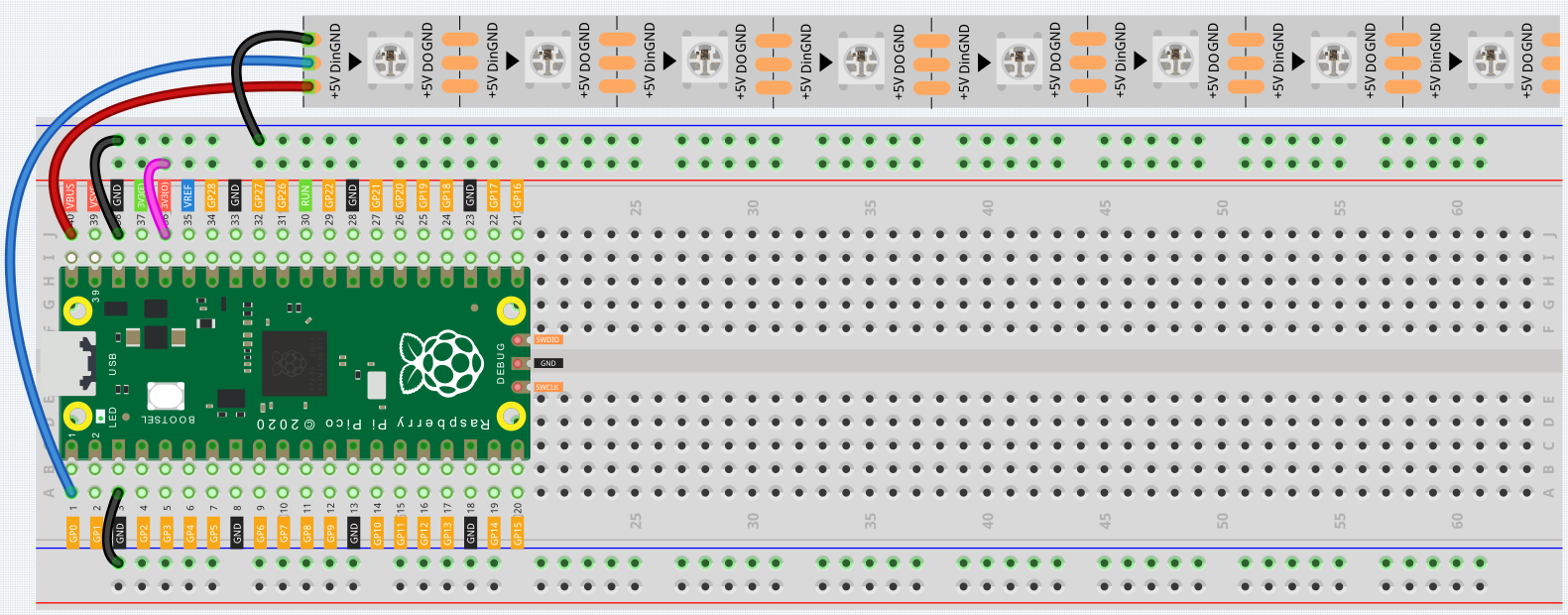# RGB LED Strip¶

WS2812 is a intelligent control LED light source that the control circuit and RGB chip are integrated in a package of 5050 components. It internal include intelligent digital port data latch and signal reshaping amplification drive circuit. Also include a precision internal oscillator and a 12V voltage programmable constant current control part, effectively ensuring the pixel point light color height consistent.

The data transfer protocol use single NZR communication mode. After the pixel power-on reset, the DIN port receive data from controller, the first pixel collect initial 24bit data then sent to the internal data latch, the other data which reshaping by the internal signal reshaping amplification circuit sent to the next cascade pixel through the DO port. After transmission for each pixel，the signal to reduce 24bit. pixel adopt auto reshaping transmit technology, making the pixel cascade number is not limited the signal transmission, only depend on the speed of signal transmission.

## Wiring¶1. Connect the +5V of the LED Strip to the VBUS of the Pico.

2. Connect the GND of the LED Strip to the GND of the Pico.

3. Connect the DIN of the LED Strip to the GP0 of Pico.

Warning

One thing you need to pay attention to is current.

Although the LED Strip with any number of LEDs can be used in Pico, the power of its VBUS pin is limited. Here, we will use eight LEDs, which are safe. But if you want to use more LEDs, you need to add a separate power supply.

## Code¶

The following is the library of ws2812 packaged by Sunfounder. You need to save it in Pico and name it as ws2812.py for use as a library.

```import array, time
import rp2
from rp2 import PIO, StateMachine, asm_pio

@asm_pio(sideset_init=PIO.OUT_LOW, out_shiftdir=PIO.SHIFT_LEFT, autopull=True, pull_thresh=24)
def ws2812():
T1 = 2
T2 = 5
T3 = 3
label("bitloop")
out(x, 1).side(0)[T3 - 1]
jmp(not_x, "do_zero").side(1)[T1 - 1]
jmp("bitloop").side(1)[T2 - 1]
label("do_zero")
nop().side(0)[T2 - 1]

class WS2812():

def __init__(self, pin, num):
# Configure the number of WS2812 LEDs.
self.led_nums = num
self.pin = pin
self.sm = StateMachine(0, ws2812, freq=8000000, sideset_base=self.pin)
# Start the StateMachine, it will wait for data on its FIFO.
self.sm.active(1)

self.buf = array.array("I", [0 for _ in range(self.led_nums)])

def write(self):
self.sm.put(self.buf, 8)

def write_all(self, value):
for i in range(self.led_nums):
self.__setitem__(i, value)
self.write()

def list_to_hex(self, color):
if isinstance(color, list) and len(color) == 3:
c = (color << 8) + (color << 16) + (color)
return c
elif isinstance(color, int):
value = (color & 0xFF0000)>>8 | (color & 0x00FF00)<<8 | (color & 0x0000FF)
return value
else:
raise ValueError("Color must be 24-bit  RGB hex or list of 3 8-bit RGB")

def hex_to_list(self, color):
if isinstance(color, list) and len(color) == 3:
return color
elif isinstance(color, int):
r = color >> 8 & 0xFF
g = color >> 16 & 0xFF
b = color >> 0 & 0xFF
return [r, g, b]
else:
raise ValueError("Color must be 24-bit  RGB hex or list of 3 8-bit RGB")

def __getitem__(self, i):
return self.hex_to_list(self.buf[i])

def __setitem__(self, i, value):
value = self.list_to_hex(value)
self.buf[i] = value
```

Then, create a new file, and call the stored ws2812 library here.

```import machine
from ws2812 import WS2812

ws = WS2812(machine.Pin(0),8)

ws = [64,154,227]
ws = [128,0,128]
ws = [50,150,50]
ws = [255,30,30]
ws = [0,128,255]
ws = [99,199,0]
ws = [128,128,128]
ws = [255,100,0]
ws.write()
```

Let’s select some favorite colors and display them on the RGB LED Strip!

## How it works?¶

In the ws2812 library, we have integrated related functions into the WS2812 class.

You can use the RGB LED Strip with the following statement.

```from ws2812 import WS2812
```

Declare a WS2812 type object, named “ws”, it is connected to “pin”, there are “number” RGB LEDs on the WS2812 strip.

```ws = WS2812(pin,number)
```

ws is an array object, each element corresponds to one RGB LED on the WS2812 strip, for example, ws is the first one, ws is the eighth.

We can assign color values to each RGB LED, these values must be 24-bit color (represented with six hexadecimal digits) or list of 3 8-bit RGB.

For example, the red value is “0xFF0000” or “[255,0,0]”.

```ws[i] = color value
```

Then use this statement to write the color for the LED Strip and light it up.

```ws.write()
```

You can also directly use the following statement to make all LEDs light up the same color.

```ws.write_all(color value)
```

## What more?¶

We can randomly generate colors and make a colorful flowing light.

```import machine
from ws2812 import WS2812
import utime
import urandom

ws = WS2812(machine.Pin(0),8)

def flowing_light():
for i in range(7,0,-1):
ws[i] = ws[i-1]
ws = int(urandom.uniform(0, 0xFFFFFF))
ws.write()
utime.sleep_ms(80)

while True:
flowing_light()
print(ws)
```